Search IntMath
Close

450+ Math Lessons written by Math Professors and Teachers

5 Million+ Students Helped Each Year

1200+ Articles Written by Math Educators and Enthusiasts

Simplifying and Teaching Math for Over 23 Years

# Constrained data model - solution(?)

By Murray Bourne, 13 Aug 2012

## Background

Reader Mike Wilde, who is 78 years old, wrote recently to say:

I have 3 inter-related formulas I developed years ago while still on the job to help me predict future expenses. I just thought you might be interested in evaluating them.

Here's what Mike was trying to do with his model (I have emphasized the main points):

My aim consisted of determining a method of smoothing out a given series of interrelated, recorded and verified data relevant to a specific, ongoing activity, no matter how erratic, over a given known period of time (population growth for example, or, in my case, the expenses pertaining to single marketing departments), given the following constraints:

(1) The sum of the single values of the resultant curve would exactly equal the sum of the single values of the original series of data.

(2) The assumption is this curve, owing to this specific characteristic, could be extended to reliably project forward into the future (provided, of course, that new, unforeseen values would not come into play after the curve had begun to move forward beyond the end value of the original, given series).

(3) I also took into account the consideration that activities of the type I had in mind could not go on moving ahead forever and must sooner or later reach a stage where there is hardly any change at all from one point to the next.

(4) This ongoing procedure would also have as an intrinsic characteristic that the sum of the first and last terms (a1 and f1 in the attached formulas) would be the maximum level of growth toward which the the curve would gradually approach, though never actually reach.

## Excel example

Mike sent me an Excel spreadsheet which demonstrates his data model.

Here's the spreadsheet: Historic Development Curve

The included data is the same set I used for my earlier challenge question, and it's there to give you an idea how the model works. You can change the data in the spreadsheet if you wish to try other scenarios.

I also included Excel's prediction (in the second chart on the spreadsheet).

## Formulas

Here are the formulas used to create the values in Column B in the spreadsheet:

n = the number of terms in a given, original series;

r = the rate of gradual growth (or decline) of the curve developed with the aid of the formulas shown above, on the basis of the series of n terms under consideration, this rate being such that the ratio between any two consecutive terms contained in the curve progressively approaches 1 and that the ratio of the difference between the 1st and 2nd, and the 2nd and 3rd terms of any three consecutive terms contained in it is always equal to this same rate of growth (or decline);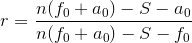a0 = the first term in the original series under consideration;

f0 = the last, or n-th term in the original series under consideration;

a1 = the first term of the curve developed by means of the formulas;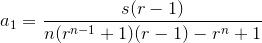f1 = the last, or n-th term of the curve developed by means of the formulas;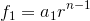S = the sum total of the n terms of the curve developed by means of the formulas, which must exactly equal the sum total of the corresponding n terms of the original series.

## The model

The formula which Mike used to give the values in Column F in the spreadsheet (the curved green line) is as follows (where n is the data number in Column D):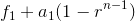First, let's point out the parts that do seem to work well.

Mike's model certainly has the first required characteristic, that the sum of the given data points is the same as the sum of the derived points. (They are both 64 in the case of the default data given.)

Considering the 3rd characteristic, that the rate of change becomes less as time goes on, is also true of the model. It tends to "flatten out" the more you project into the future.

Now for the 4th characteristic, that the sum a1 + f1 gives the limiting value of the model. This is certainly true for the default 10 data points I have incldued in the spreadsheet. This is a consequence of the model's formula,As n gets larger, the rn−1 term gets smaller and eventually will disappear.

Now, the default data I used has a logarithmic-shaped curve.

Here's the original data and the values given by the model:

 t s m 1 2 3 4 5 6 7 8 9 10 Σ 2 4 3 5 9 6 8 9 8 10 64 2.17 3.53 4.69 5.68 6.52 7.23 7.84 8.36 8.8 9.18 64

Here's the graph of the original data (in gray) and the model (in green), extrapolated to t = 30: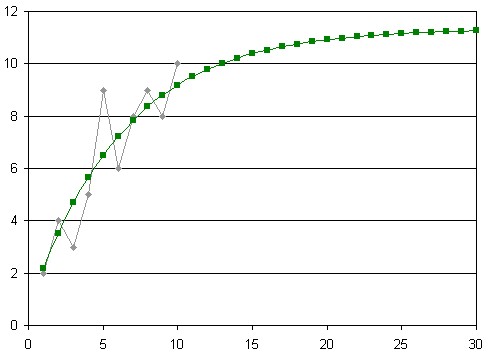We can see it produces quite a good fit and the predicted values are reasonable given the shape of the original data.

## Problems

Unfortunately, any data with a significantly different shape will produce unconvincing results.

For example, let's try this data, which has an (approximately) exponentially decreasing shape:

 t s 1 2 3 4 5 6 7 8 9 10 10 8 6 5.5 5 4.6 4.3 4.1 4 3.95

We can see from the table this data is trending to a value just under 4 and flat-lining.

The model now behaves strangely, giving us the following when we extrapolate out to t = 30: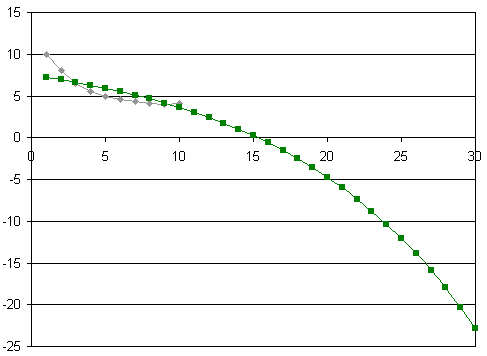The curvature is not correct (the model is concave down, while the original data is concave up) and it has not picked up the levelling out of the original data (something which the model claims to do).

Also, if the data represented sales figures (for example), it would be strange to get negative values like this!

For interest, I used Excel's "Add trendline" feature and extrapolated to t = 30. It gives a more convincing model.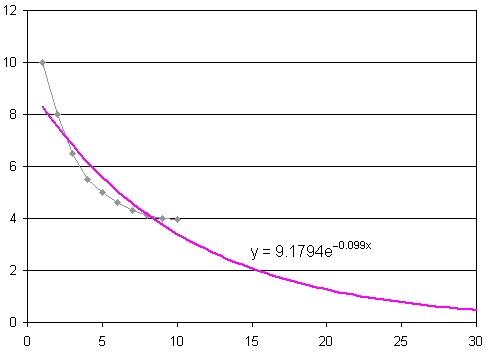Something to note here is that the sum of my data points is 55.95. Using the Excel trendline formula shown on the chart for the values from 1 to 10 gives the following (marked as "E" in the table, with the original values for comparison):

 t s E 1 2 3 4 5 6 7 8 9 10 10 8 6 5.55 5 4.6 4.3 4.1 4 3.95 8.3 7.5 6.8 6.2 5.6 5.1 4.6 4.2 3.8 3.4

The sum of Excel's values is 55.43 which is very close to the sum of the data values.

I tried several other graph shapes and obtained similar misleading results.

For another example, if you input linear data, the model "flatlines", like this (where we would reasonably expect the predicted values to continue in a linear fashion):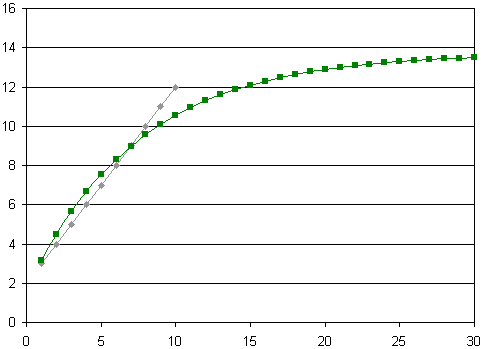To be fair, the above example is following the main constraints given, that the growth will decrease over time and tend towards some fixed value, and the sum of the given 10 data values is the same as the sum of the first model's first 10 values (23 in this case).

Also, quite a few of the data sets I tried resulted in Division by 0 errors.

So my conclusion is the model seems to work quite well for data that gives a logarithmically-shaped curve (steeply rising to begin with, then flattening out as time goes on), but tends to fall over for other data types.

I suspect Mike's data sets were logarithmic in nature so he found the model to be useful for his work-related tasks.

## Original reasoning

Unfortunately, Mike's original rationale for his model is lost. He wrote:

As to how I derived the formulas, all my notes, jottings and whatnot have disappeared into the mists of time (I first did them some 20 years ago).

The formulas are mine and mine alone and are based on knowledge gained when a high school student much longer ago and further away than I care to contemplate.

## Final notes

Thanks for sharing your model, Mike!

This means we still don't have a solution for my earlier challenge. There's probably a proof somewhere that says it can't be done (for any shaped data set). But I'd sure like to think it can be done!

Be the first to comment below.

### Comment Preview

HTML: You can use simple tags like <b>, <a href="...">, etc.

To enter math, you can can either:

1. Use simple calculator-like input in the following format (surround your math in backticks, or qq on tablet or phone):
a^2 = sqrt(b^2 + c^2)
(See more on ASCIIMath syntax); or
2. Use simple LaTeX in the following format. Surround your math with $$ and $$.
$$\int g dx = \sqrt{\frac{a}{b}}$$
(This is standard simple LaTeX.)

NOTE: You can mix both types of math entry in your comment.